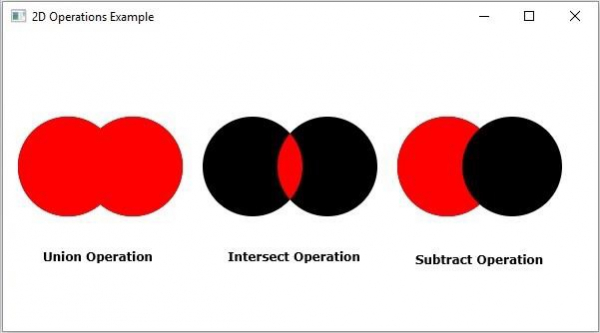# What are various operations of 2D objects in JavaFX?

JavaFX supports three operations on 2D objects namely – Union, Subtraction and Intersection.

• Union Operation − This operation takes two or more shapes as inputs and returns the area occupied by them.

• Intersection Operation − This operation takes two or more shapes as inputs and returns the intersection area between them.

• Subtraction Operation − This operation takes two or more shapes as an input. Then, it returns the area of the first shape excluding the area overlapped by the second one.

## Example

import javafx.application.Application;
import javafx.scene.Group;
import javafx.scene.Scene;
import javafx.scene.paint.Color;
import javafx.stage.Stage;
import javafx.scene.shape.Circle;
import javafx.scene.shape.Shape;
import javafx.scene.text.Font;
import javafx.scene.text.FontPosture;
import javafx.scene.text.FontWeight;
import javafx.scene.text.Text;
public class JavaFXOperations extends Application {
public void start(Stage stage) {
Font font = Font.font("verdana", FontWeight.BOLD, FontPosture.REGULAR, 12);
//Drawing circles for union operation
Circle shape1 = new Circle(65.0f, 135.0f, 50.0f);
Circle shape2 = new Circle(130.0f, 135.0f, 50.0f );
//Union operation
Shape union = Shape.union(shape1, shape2);
union.setFill(Color.RED);
Text label1 = new Text("Union Operation");
label1.setFont(font);
label1.setX(40);
label1.setY(230);
//Drawing circles for union operation
Circle shape3 = new Circle(250.0f, 135.0f, 50.0f);
Circle shape4 = new Circle(325.0f, 135.0f, 50.0f );
//Intersect operation
Shape intersect = Shape.intersect(shape3, shape4);
intersect.setFill(Color.RED);
Text label2 = new Text("Intersect Operation");
label2.setFont(font);
label2.setX(225);
label2.setY(230);
//Drawing circles for union operation
Circle shape5 = new Circle(445.0f, 135.0f, 50.0f);
Circle shape6 = new Circle(510.0f, 135.0f, 50.0f );
//Union operation
Shape subtract = Shape.subtract(shape5, shape6);
subtract.setFill(Color.RED);
Text label3 = new Text("Subtract Operation");
label3.setFont(font);
label3.setX(420);
label3.setY(230);
//Setting the stage
Group root = new Group(
shape1, shape2, shape3, shape4, shape5, shape6,
union, intersect, subtract, label1, label2, label3
);
Scene scene = new Scene(root, 600, 300);
stage.setTitle("2D Operations Example");
stage.setScene(scene);
stage.show();
}
public static void main(String args[]){
launch(args);
}
}

## Output